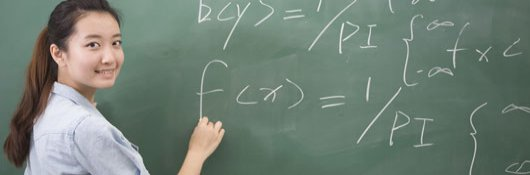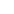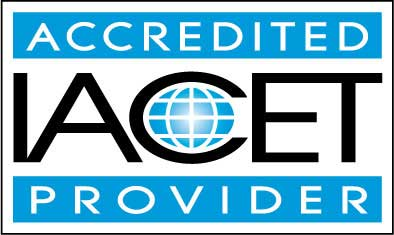# Course Syllabus## Course Description

Calculus is a mathematical tool that is required in a variety of scientific, technical, and other professional fields. Because it is a higher-level area of math, many people think it is a subject that they can never understand. Unfortunately, such an attitude limits an individual's career choices, particularly in a technology-focused society.

This course provides a bridge between algebra and calculus, guiding students through the underlying mathematical concepts needed to understand and appreciate calculus. The course reviews real and complex numbers, functions, and equations and inequalities. It then goes into some detail on specific types of functions, such as polynomials, exponentials, and logarithms. It also provides a derivation of the principles of trigonometry on the basis of simple right-triangle and circle geometry. After reviewing vectors, polar coordinates, and conic sections, the material sets up calculus proper by examining limits as well as sequences and series.

The final lessons of the course use the basic principles of algebra to derive the two key tools of calculus: differentiation (the derivative) and integration (the integral). At the end of this course, students will be prepared to study calculus in greater depth, further investigating and applying derivatives and integrals to solve a variety of math, physics, and other problems.

• Completely Online
• Self-Paced
• Printable Lessons
• Full HD Video• 6 Months to Complete
• Start Anytime
• PC & Mac Compatible
• Android & iOS Friendly
• Accredited CEUs## Learning Outcomes

By successfully completing this course, students will be able to:
• Define, identify and demonstrate use of real and complex numbers.
• Define, identify and demonstrate use of functions.
• Define, identify and demonstrate use of equations and inequalities.
• Define, identify and demonstrate use of polynomials and rational functions.
• Define, identify and demonstrate use of exponential and logarithmic functions.
• Define, identify and demonstrate use of trigonometric functions.
• Define, identify and demonstrate use of vectors.
• Define, identify and demonstrate use of polar coordinates.
• Define, identify and demonstrate use of conic sections.
• Define, identify and demonstrate use of limits, sequences, and series.
• Define, identify and demonstrate use of derivatives and integrals.
• Demonstrate mastery of lesson content at levels of 70% or higher.

## Assessment Guide

Assessment Points
Introduction 1 points
Lesson 1 Exam 20 points
Lesson 2 Exam 20 points
Lesson 3 Exam 20 points
Lesson 4 Exam 20 points
Lesson 5 Exam 20 points
Lesson 6 Exam 20 points
Lesson 7 Exam 20 points
Lesson 8 Exam 20 points
Lesson 9 Exam 20 points
Lesson 10 Exam 20 points
Lesson 11 Exam 20 points
Lesson 12 Exam 20 points
Lesson 13 Exam 20 points
Lesson 14 Exam 20 points
Lesson 15 Exam 20 points
Lesson 16 Exam 20 points
Lesson 17 Exam 20 points
Lesson 18 Exam 20 points
Lesson 19 Exam 20 points
The Final Exam 80 points